Скачать презентацию PART V THE GOODS AND MONEY MARKETS Chapter

• Количество слайдов: 34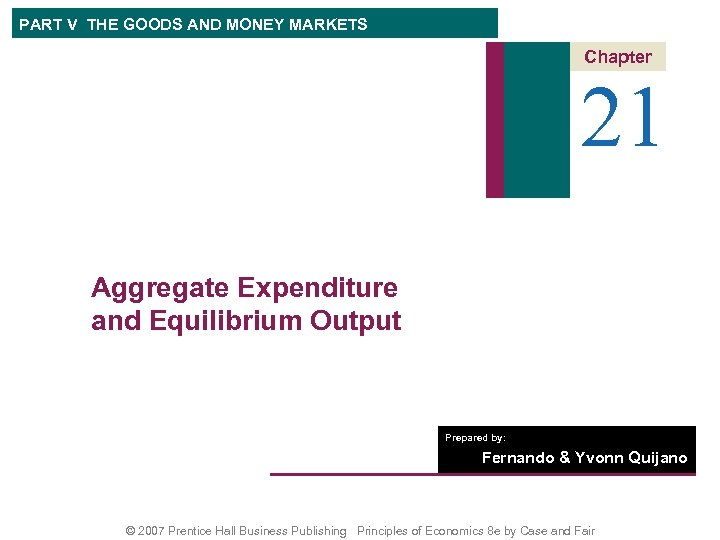PART V THE GOODS AND MONEY MARKETS Chapter 21 Aggregate Expenditure and Equilibrium Output Prepared by: Fernando & Yvonn Quijano © 2007 Prentice Hall Business Publishing Principles of Economics 8 e by Case and Fair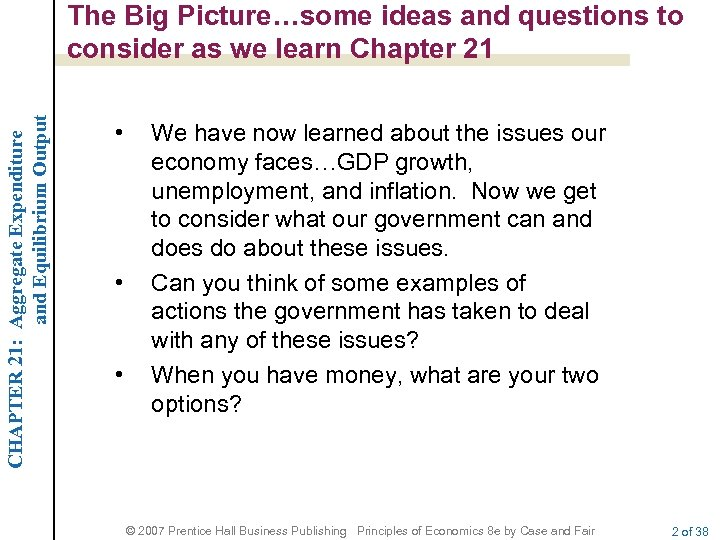CHAPTER 21: Aggregate Expenditure and Equilibrium Output The Big Picture…some ideas and questions to consider as we learn Chapter 21 • • • We have now learned about the issues our economy faces…GDP growth, unemployment, and inflation. Now we get to consider what our government can and does do about these issues. Can you think of some examples of actions the government has taken to deal with any of these issues? When you have money, what are your two options? © 2007 Prentice Hall Business Publishing Principles of Economics 8 e by Case and Fair 2 of 38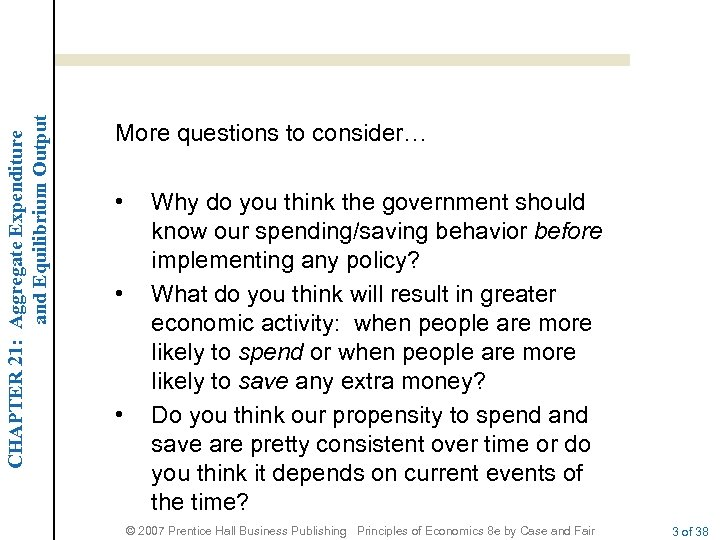CHAPTER 21: Aggregate Expenditure and Equilibrium Output More questions to consider… • • • Why do you think the government should know our spending/saving behavior before implementing any policy? What do you think will result in greater economic activity: when people are more likely to spend or when people are more likely to save any extra money? Do you think our propensity to spend and save are pretty consistent over time or do you think it depends on current events of the time? © 2007 Prentice Hall Business Publishing Principles of Economics 8 e by Case and Fair 3 of 38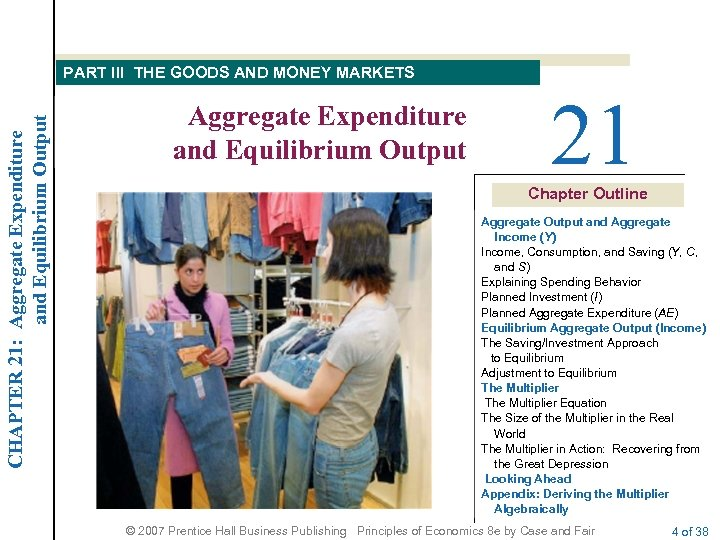CHAPTER 21: Aggregate Expenditure and Equilibrium Output PART III THE GOODS AND MONEY MARKETS Aggregate Expenditure and Equilibrium Output 21 Chapter Outline Aggregate Output and Aggregate Income (Y) Income, Consumption, and Saving (Y, C, and S) Explaining Spending Behavior Planned Investment (I) Planned Aggregate Expenditure (AE) Equilibrium Aggregate Output (Income) The Saving/Investment Approach to Equilibrium Adjustment to Equilibrium The Multiplier Equation The Size of the Multiplier in the Real World The Multiplier in Action: Recovering from the Great Depression Looking Ahead Appendix: Deriving the Multiplier Algebraically © 2007 Prentice Hall Business Publishing Principles of Economics 8 e by Case and Fair 4 of 38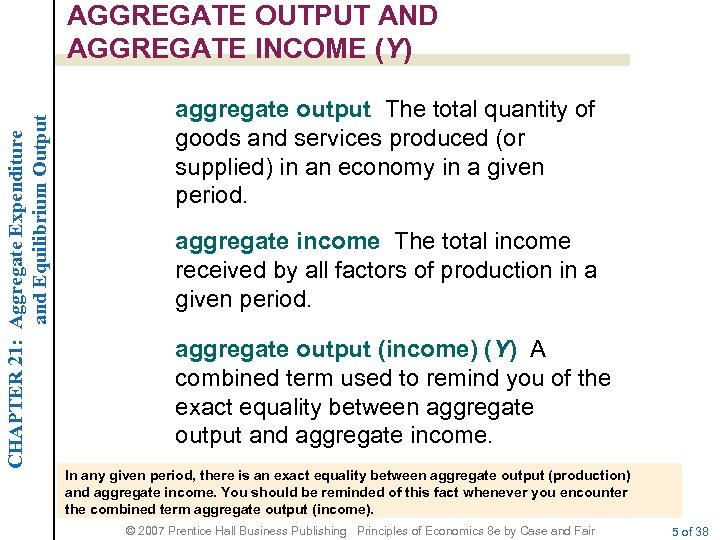CHAPTER 21: Aggregate Expenditure and Equilibrium Output AGGREGATE OUTPUT AND AGGREGATE INCOME (Y) aggregate output The total quantity of goods and services produced (or supplied) in an economy in a given period. aggregate income The total income received by all factors of production in a given period. aggregate output (income) (Y) A combined term used to remind you of the exact equality between aggregate output and aggregate income. In any given period, there is an exact equality between aggregate output (production) and aggregate income. You should be reminded of this fact whenever you encounter the combined term aggregate output (income). © 2007 Prentice Hall Business Publishing Principles of Economics 8 e by Case and Fair 5 of 38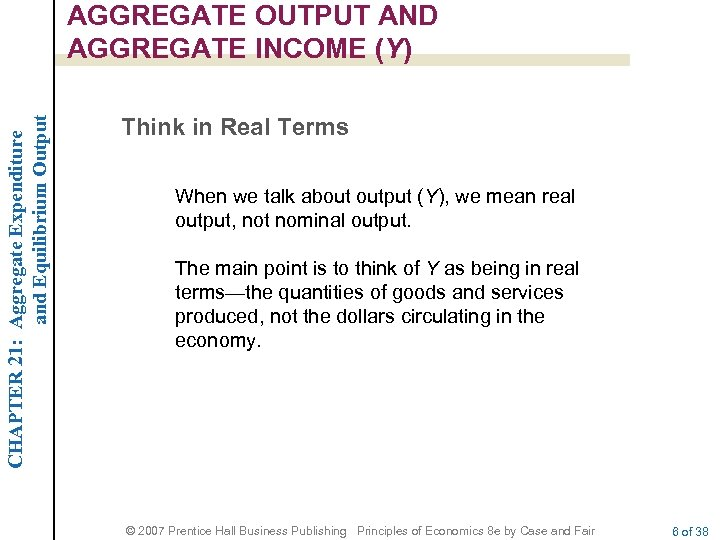CHAPTER 21: Aggregate Expenditure and Equilibrium Output AGGREGATE OUTPUT AND AGGREGATE INCOME (Y) Think in Real Terms When we talk about output (Y), we mean real output, not nominal output. The main point is to think of Y as being in real terms—the quantities of goods and services produced, not the dollars circulating in the economy. © 2007 Prentice Hall Business Publishing Principles of Economics 8 e by Case and Fair 6 of 38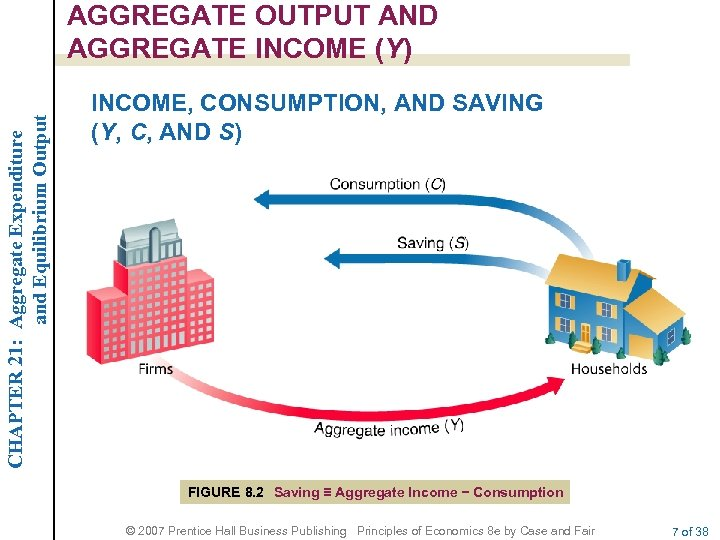CHAPTER 21: Aggregate Expenditure and Equilibrium Output AGGREGATE OUTPUT AND AGGREGATE INCOME (Y) INCOME, CONSUMPTION, AND SAVING (Y, C, AND S) FIGURE 8. 2 Saving ≡ Aggregate Income − Consumption © 2007 Prentice Hall Business Publishing Principles of Economics 8 e by Case and Fair 7 of 38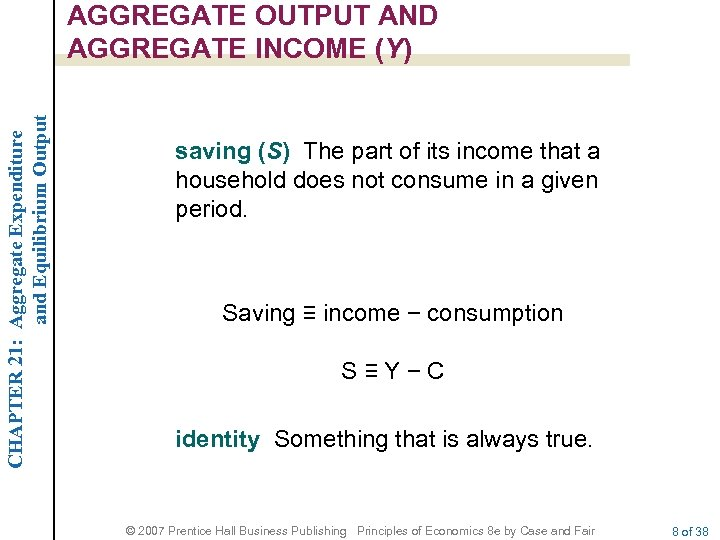CHAPTER 21: Aggregate Expenditure and Equilibrium Output AGGREGATE OUTPUT AND AGGREGATE INCOME (Y) saving (S) The part of its income that a household does not consume in a given period. Saving ≡ income − consumption S≡Y−C identity Something that is always true. © 2007 Prentice Hall Business Publishing Principles of Economics 8 e by Case and Fair 8 of 38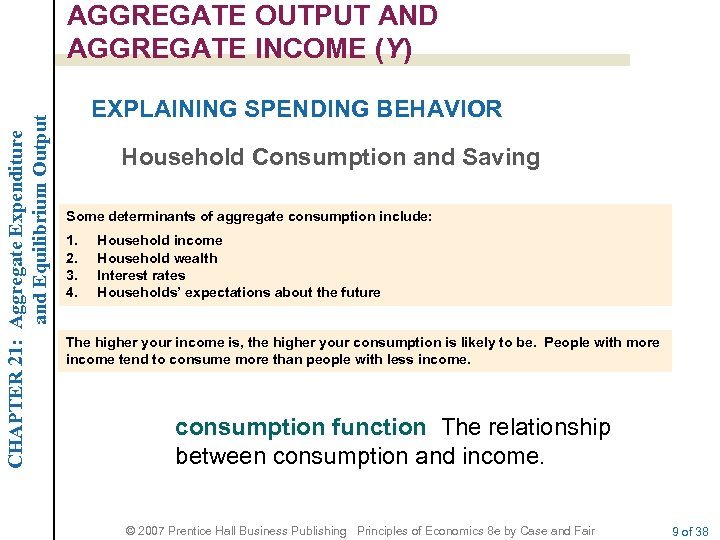CHAPTER 21: Aggregate Expenditure and Equilibrium Output AGGREGATE OUTPUT AND AGGREGATE INCOME (Y) EXPLAINING SPENDING BEHAVIOR Household Consumption and Saving Some determinants of aggregate consumption include: 1. 2. 3. 4. Household income Household wealth Interest rates Households’ expectations about the future The higher your income is, the higher your consumption is likely to be. People with more income tend to consume more than people with less income. consumption function The relationship between consumption and income. © 2007 Prentice Hall Business Publishing Principles of Economics 8 e by Case and Fair 9 of 38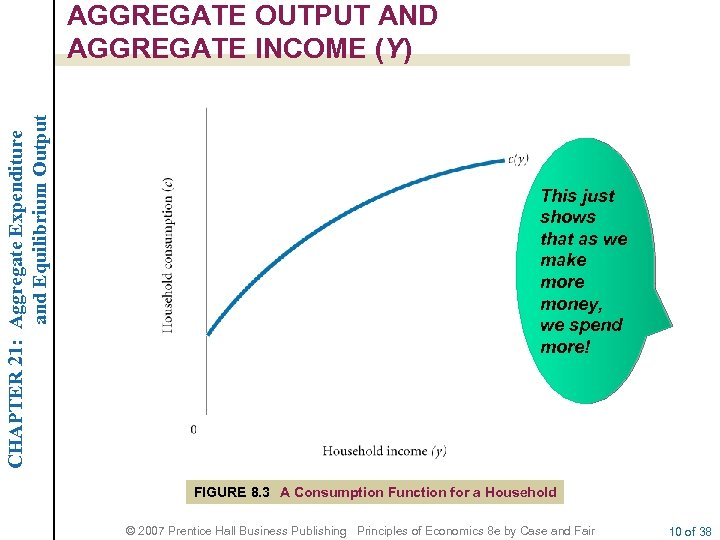CHAPTER 21: Aggregate Expenditure and Equilibrium Output AGGREGATE OUTPUT AND AGGREGATE INCOME (Y) This just shows that as we make more money, we spend more! FIGURE 8. 3 A Consumption Function for a Household © 2007 Prentice Hall Business Publishing Principles of Economics 8 e by Case and Fair 10 of 38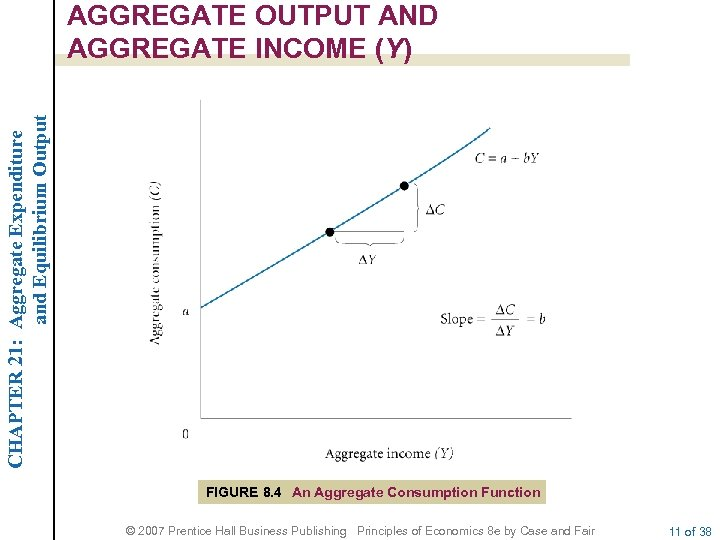CHAPTER 21: Aggregate Expenditure and Equilibrium Output AGGREGATE OUTPUT AND AGGREGATE INCOME (Y) FIGURE 8. 4 An Aggregate Consumption Function © 2007 Prentice Hall Business Publishing Principles of Economics 8 e by Case and Fair 11 of 38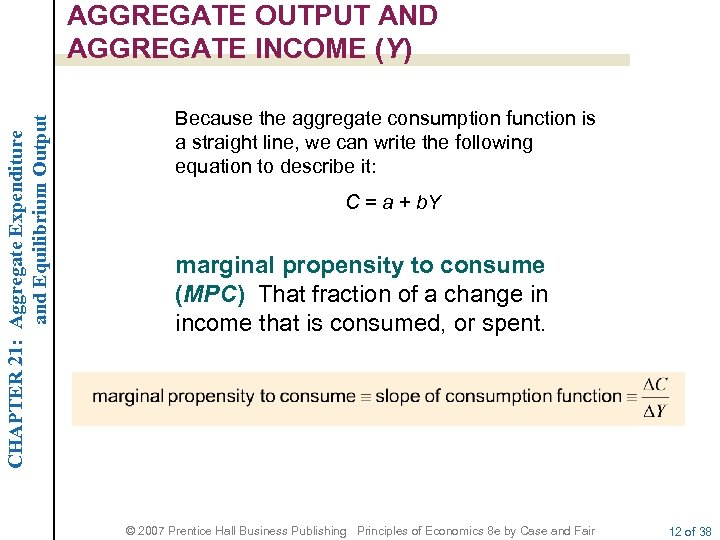CHAPTER 21: Aggregate Expenditure and Equilibrium Output AGGREGATE OUTPUT AND AGGREGATE INCOME (Y) Because the aggregate consumption function is a straight line, we can write the following equation to describe it: C = a + b. Y marginal propensity to consume (MPC) That fraction of a change in income that is consumed, or spent. © 2007 Prentice Hall Business Publishing Principles of Economics 8 e by Case and Fair 12 of 38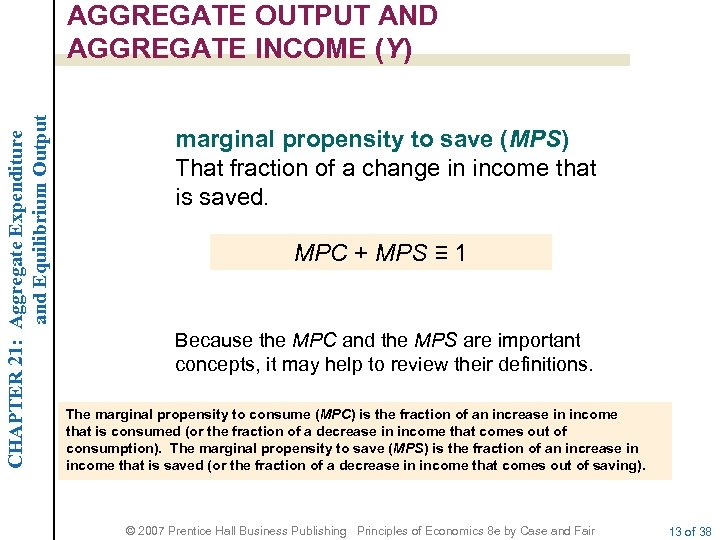CHAPTER 21: Aggregate Expenditure and Equilibrium Output AGGREGATE OUTPUT AND AGGREGATE INCOME (Y) marginal propensity to save (MPS) That fraction of a change in income that is saved. MPC + MPS ≡ 1 Because the MPC and the MPS are important concepts, it may help to review their definitions. The marginal propensity to consume (MPC) is the fraction of an increase in income that is consumed (or the fraction of a decrease in income that comes out of consumption). The marginal propensity to save (MPS) is the fraction of an increase in income that is saved (or the fraction of a decrease in income that comes out of saving). © 2007 Prentice Hall Business Publishing Principles of Economics 8 e by Case and Fair 13 of 38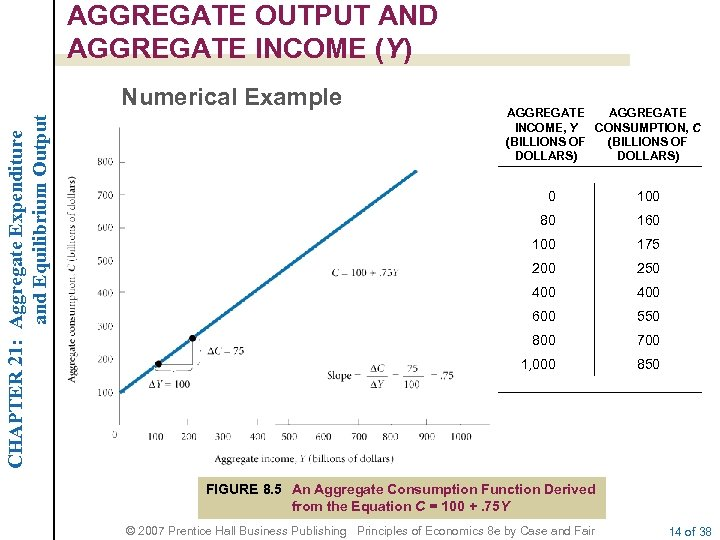AGGREGATE OUTPUT AND AGGREGATE INCOME (Y) CHAPTER 21: Aggregate Expenditure and Equilibrium Output Numerical Example AGGREGATE INCOME, Y CONSUMPTION, C (BILLIONS OF DOLLARS) 0 100 80 160 100 175 200 250 400 600 550 800 700 1, 000 850 FIGURE 8. 5 An Aggregate Consumption Function Derived from the Equation C = 100 +. 75 Y © 2007 Prentice Hall Business Publishing Principles of Economics 8 e by Case and Fair 14 of 38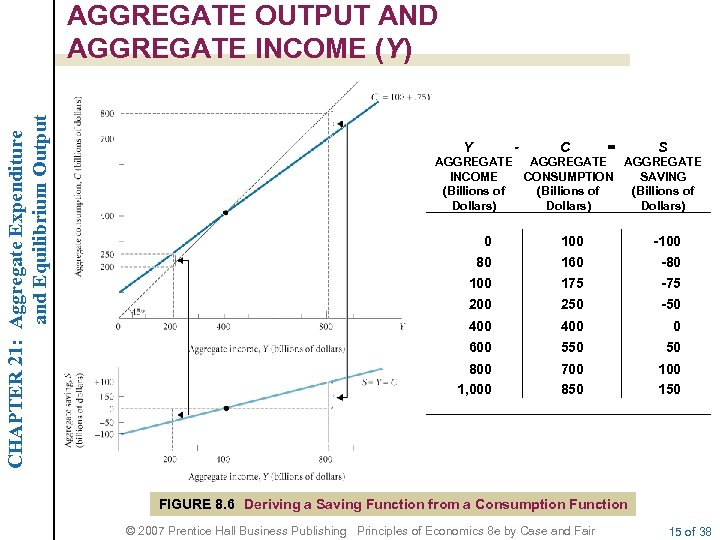CHAPTER 21: Aggregate Expenditure and Equilibrium Output AGGREGATE OUTPUT AND AGGREGATE INCOME (Y) Y - C = S AGGREGATE INCOME CONSUMPTION SAVING (Billions of Dollars) 0 80 100 160 -100 -80 100 175 -75 200 250 -50 400 0 600 550 50 800 1, 000 700 850 100 150 FIGURE 8. 6 Deriving a Saving Function from a Consumption Function © 2007 Prentice Hall Business Publishing Principles of Economics 8 e by Case and Fair 15 of 38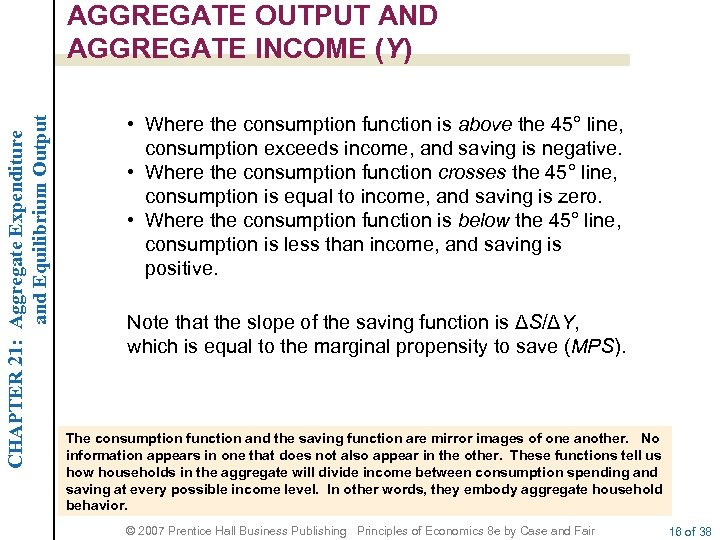CHAPTER 21: Aggregate Expenditure and Equilibrium Output AGGREGATE OUTPUT AND AGGREGATE INCOME (Y) • Where the consumption function is above the 45° line, consumption exceeds income, and saving is negative. • Where the consumption function crosses the 45° line, consumption is equal to income, and saving is zero. • Where the consumption function is below the 45° line, consumption is less than income, and saving is positive. Note that the slope of the saving function is ΔS/ΔY, which is equal to the marginal propensity to save (MPS). The consumption function and the saving function are mirror images of one another. No information appears in one that does not also appear in the other. These functions tell us how households in the aggregate will divide income between consumption spending and saving at every possible income level. In other words, they embody aggregate household behavior. © 2007 Prentice Hall Business Publishing Principles of Economics 8 e by Case and Fair 16 of 38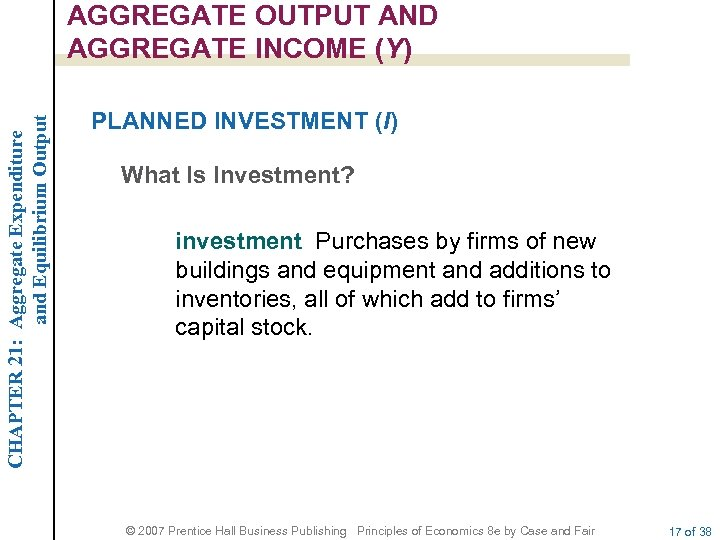CHAPTER 21: Aggregate Expenditure and Equilibrium Output AGGREGATE OUTPUT AND AGGREGATE INCOME (Y) PLANNED INVESTMENT (I) What Is Investment? investment Purchases by firms of new buildings and equipment and additions to inventories, all of which add to firms’ capital stock. © 2007 Prentice Hall Business Publishing Principles of Economics 8 e by Case and Fair 17 of 38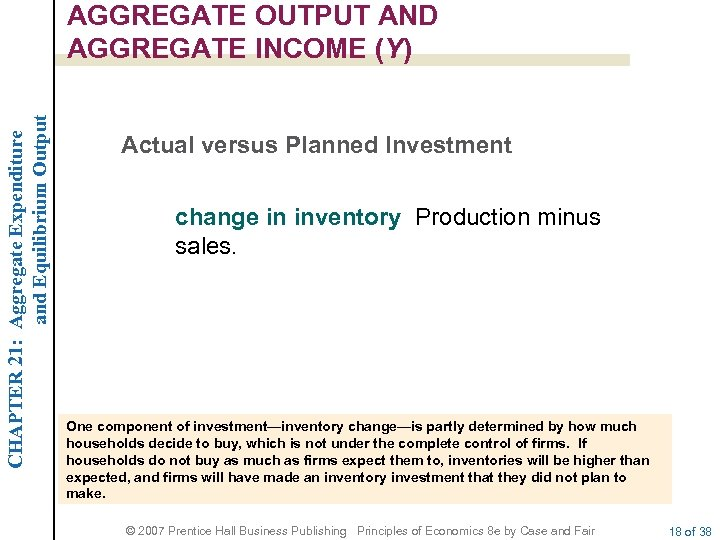CHAPTER 21: Aggregate Expenditure and Equilibrium Output AGGREGATE OUTPUT AND AGGREGATE INCOME (Y) Actual versus Planned Investment change in inventory Production minus sales. One component of investment—inventory change—is partly determined by how much households decide to buy, which is not under the complete control of firms. If households do not buy as much as firms expect them to, inventories will be higher than expected, and firms will have made an inventory investment that they did not plan to make. © 2007 Prentice Hall Business Publishing Principles of Economics 8 e by Case and Fair 18 of 38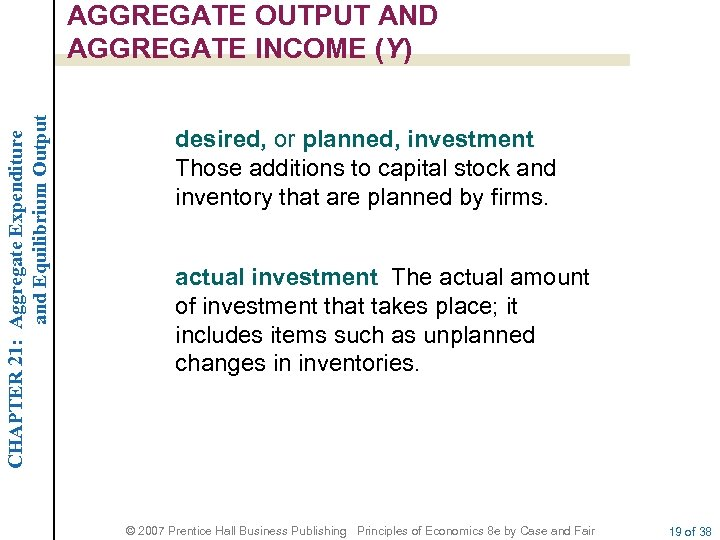CHAPTER 21: Aggregate Expenditure and Equilibrium Output AGGREGATE OUTPUT AND AGGREGATE INCOME (Y) desired, or planned, investment Those additions to capital stock and inventory that are planned by firms. actual investment The actual amount of investment that takes place; it includes items such as unplanned changes in inventories. © 2007 Prentice Hall Business Publishing Principles of Economics 8 e by Case and Fair 19 of 38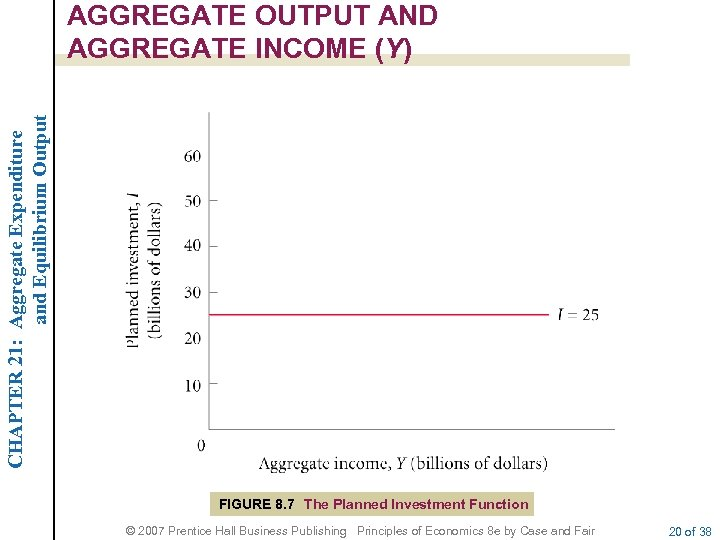CHAPTER 21: Aggregate Expenditure and Equilibrium Output AGGREGATE OUTPUT AND AGGREGATE INCOME (Y) FIGURE 8. 7 The Planned Investment Function © 2007 Prentice Hall Business Publishing Principles of Economics 8 e by Case and Fair 20 of 38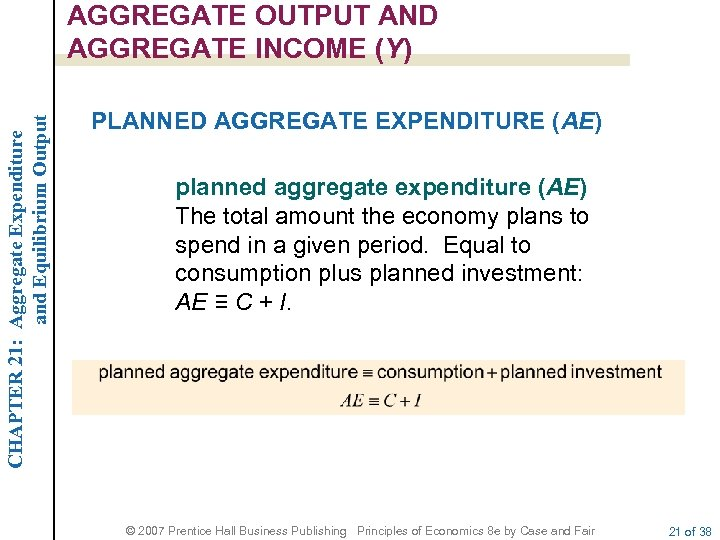CHAPTER 21: Aggregate Expenditure and Equilibrium Output AGGREGATE OUTPUT AND AGGREGATE INCOME (Y) PLANNED AGGREGATE EXPENDITURE (AE) planned aggregate expenditure (AE) The total amount the economy plans to spend in a given period. Equal to consumption plus planned investment: AE ≡ C + I. © 2007 Prentice Hall Business Publishing Principles of Economics 8 e by Case and Fair 21 of 38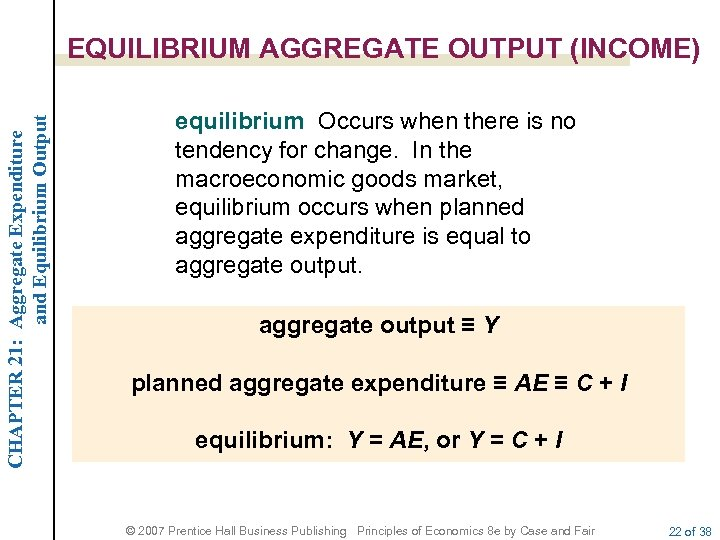CHAPTER 21: Aggregate Expenditure and Equilibrium Output EQUILIBRIUM AGGREGATE OUTPUT (INCOME) equilibrium Occurs when there is no tendency for change. In the macroeconomic goods market, equilibrium occurs when planned aggregate expenditure is equal to aggregate output ≡ Y planned aggregate expenditure ≡ AE ≡ C + I equilibrium: Y = AE, or Y = C + I © 2007 Prentice Hall Business Publishing Principles of Economics 8 e by Case and Fair 22 of 38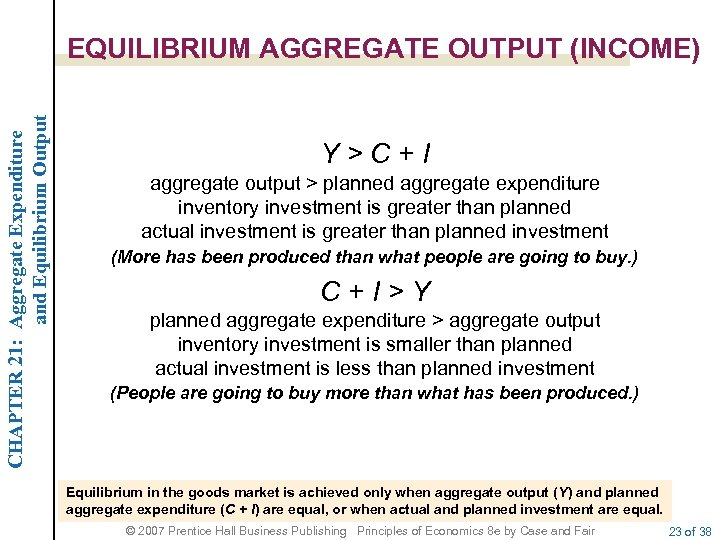CHAPTER 21: Aggregate Expenditure and Equilibrium Output EQUILIBRIUM AGGREGATE OUTPUT (INCOME) Y>C+I aggregate output > planned aggregate expenditure inventory investment is greater than planned actual investment is greater than planned investment (More has been produced than what people are going to buy. ) C+I>Y planned aggregate expenditure > aggregate output inventory investment is smaller than planned actual investment is less than planned investment (People are going to buy more than what has been produced. ) Equilibrium in the goods market is achieved only when aggregate output (Y) and planned aggregate expenditure (C + I) are equal, or when actual and planned investment are equal. © 2007 Prentice Hall Business Publishing Principles of Economics 8 e by Case and Fair 23 of 38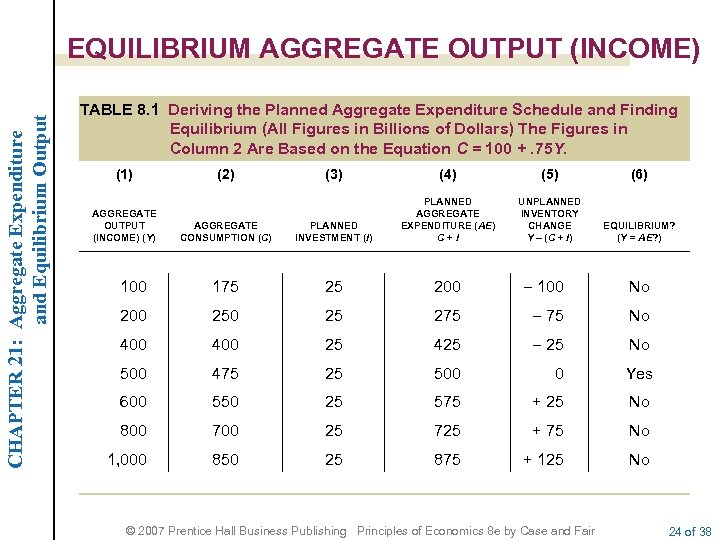CHAPTER 21: Aggregate Expenditure and Equilibrium Output EQUILIBRIUM AGGREGATE OUTPUT (INCOME) TABLE 8. 1 Deriving the Planned Aggregate Expenditure Schedule and Finding Equilibrium (All Figures in Billions of Dollars) The Figures in Column 2 Are Based on the Equation C = 100 +. 75 Y. (1) (3) (4) (5) (6) AGGREGATE CONSUMPTION (C) PLANNED INVESTMENT (I) PLANNED AGGREGATE EXPENDITURE (AE) C+I UNPLANNED INVENTORY CHANGE Y - (C + I) EQUILIBRIUM? (Y = AE? ) 100 175 25 200 - 100 No 200 25 275 - 75 No 400 25 425 - 25 No 500 475 25 500 0 Yes 600 550 25 575 + 25 No 800 700 25 725 + 75 No 1, 000 850 25 875 + 125 No AGGREGATE OUTPUT (INCOME) (Y) (2) © 2007 Prentice Hall Business Publishing Principles of Economics 8 e by Case and Fair 24 of 38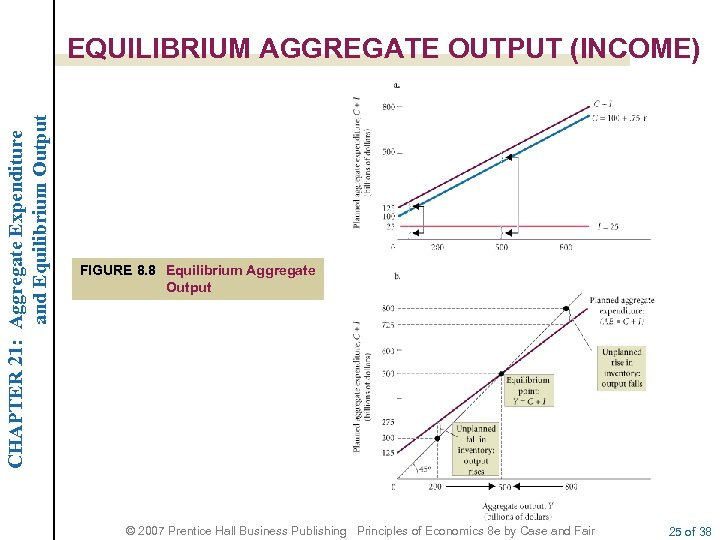CHAPTER 21: Aggregate Expenditure and Equilibrium Output EQUILIBRIUM AGGREGATE OUTPUT (INCOME) FIGURE 8. 8 Equilibrium Aggregate Output © 2007 Prentice Hall Business Publishing Principles of Economics 8 e by Case and Fair 25 of 38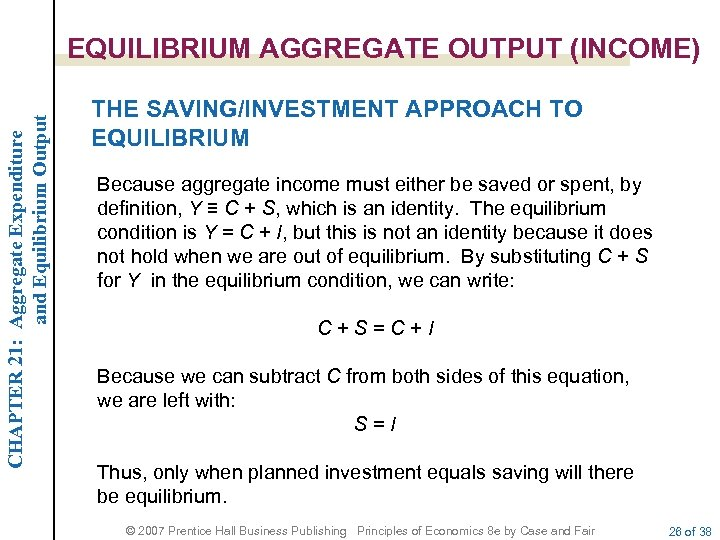CHAPTER 21: Aggregate Expenditure and Equilibrium Output EQUILIBRIUM AGGREGATE OUTPUT (INCOME) THE SAVING/INVESTMENT APPROACH TO EQUILIBRIUM Because aggregate income must either be saved or spent, by definition, Y ≡ C + S, which is an identity. The equilibrium condition is Y = C + I, but this is not an identity because it does not hold when we are out of equilibrium. By substituting C + S for Y in the equilibrium condition, we can write: C+S=C+I Because we can subtract C from both sides of this equation, we are left with: S=I Thus, only when planned investment equals saving will there be equilibrium. © 2007 Prentice Hall Business Publishing Principles of Economics 8 e by Case and Fair 26 of 38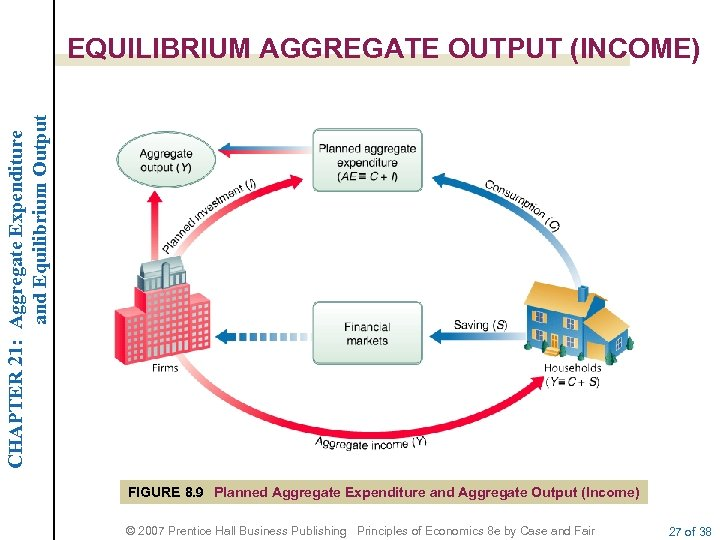CHAPTER 21: Aggregate Expenditure and Equilibrium Output EQUILIBRIUM AGGREGATE OUTPUT (INCOME) FIGURE 8. 9 Planned Aggregate Expenditure and Aggregate Output (Income) © 2007 Prentice Hall Business Publishing Principles of Economics 8 e by Case and Fair 27 of 38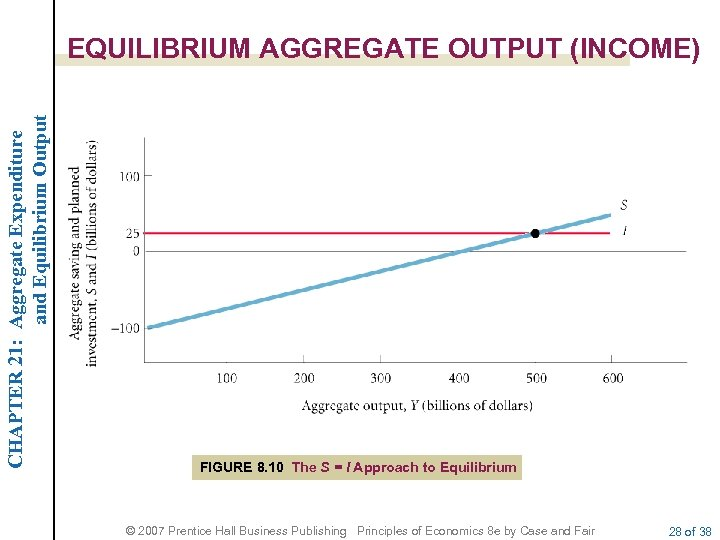CHAPTER 21: Aggregate Expenditure and Equilibrium Output EQUILIBRIUM AGGREGATE OUTPUT (INCOME) FIGURE 8. 10 The S = I Approach to Equilibrium © 2007 Prentice Hall Business Publishing Principles of Economics 8 e by Case and Fair 28 of 38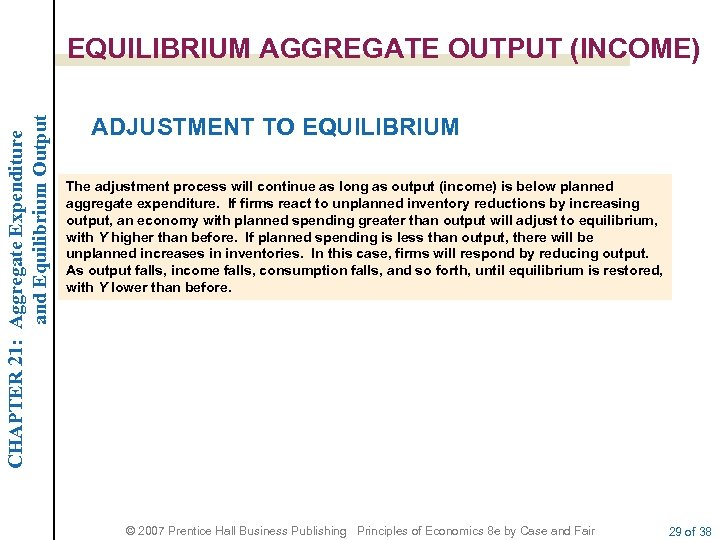CHAPTER 21: Aggregate Expenditure and Equilibrium Output EQUILIBRIUM AGGREGATE OUTPUT (INCOME) ADJUSTMENT TO EQUILIBRIUM The adjustment process will continue as long as output (income) is below planned aggregate expenditure. If firms react to unplanned inventory reductions by increasing output, an economy with planned spending greater than output will adjust to equilibrium, with Y higher than before. If planned spending is less than output, there will be unplanned increases in inventories. In this case, firms will respond by reducing output. As output falls, income falls, consumption falls, and so forth, until equilibrium is restored, with Y lower than before. © 2007 Prentice Hall Business Publishing Principles of Economics 8 e by Case and Fair 29 of 38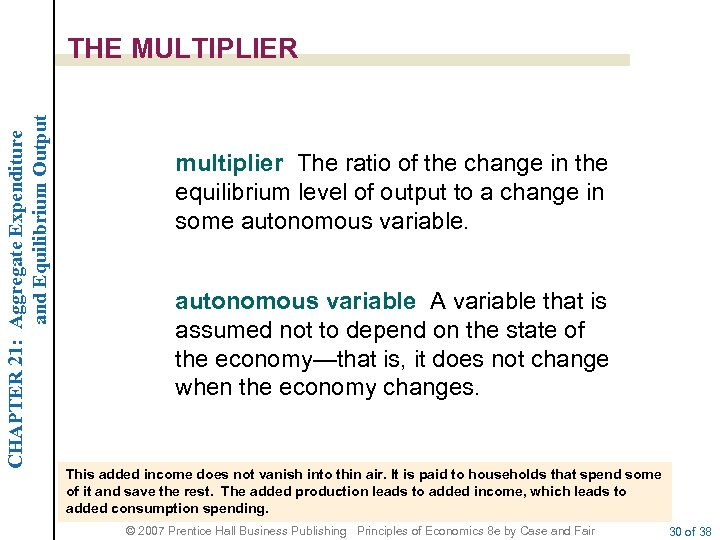CHAPTER 21: Aggregate Expenditure and Equilibrium Output THE MULTIPLIER multiplier The ratio of the change in the equilibrium level of output to a change in some autonomous variable A variable that is assumed not to depend on the state of the economy—that is, it does not change when the economy changes. This added income does not vanish into thin air. It is paid to households that spend some of it and save the rest. The added production leads to added income, which leads to added consumption spending. © 2007 Prentice Hall Business Publishing Principles of Economics 8 e by Case and Fair 30 of 38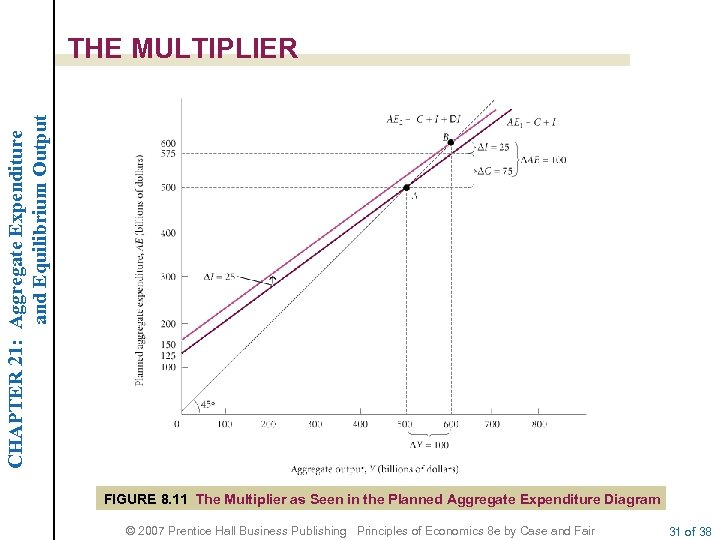CHAPTER 21: Aggregate Expenditure and Equilibrium Output THE MULTIPLIER FIGURE 8. 11 The Multiplier as Seen in the Planned Aggregate Expenditure Diagram © 2007 Prentice Hall Business Publishing Principles of Economics 8 e by Case and Fair 31 of 38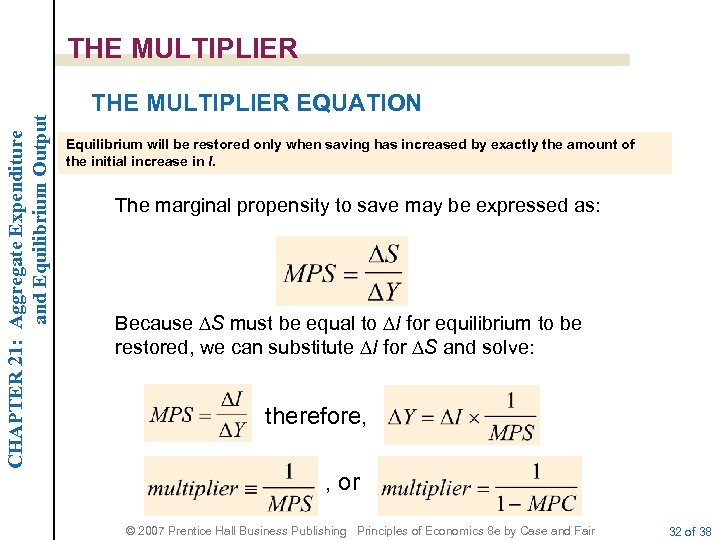CHAPTER 21: Aggregate Expenditure and Equilibrium Output THE MULTIPLIER EQUATION Equilibrium will be restored only when saving has increased by exactly the amount of the initial increase in I. The marginal propensity to save may be expressed as: Because DS must be equal to DI for equilibrium to be restored, we can substitute DI for DS and solve: therefore, , or © 2007 Prentice Hall Business Publishing Principles of Economics 8 e by Case and Fair 32 of 38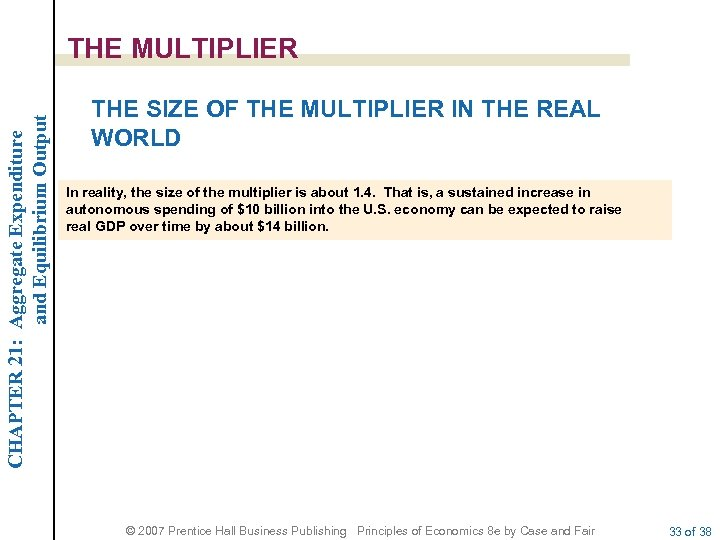CHAPTER 21: Aggregate Expenditure and Equilibrium Output THE MULTIPLIER THE SIZE OF THE MULTIPLIER IN THE REAL WORLD In reality, the size of the multiplier is about 1. 4. That is, a sustained increase in autonomous spending of \$10 billion into the U. S. economy can be expected to raise real GDP over time by about \$14 billion. © 2007 Prentice Hall Business Publishing Principles of Economics 8 e by Case and Fair 33 of 38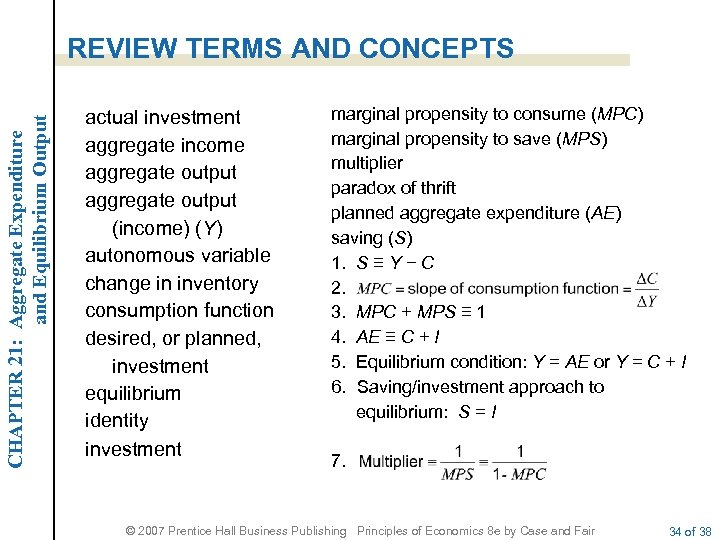CHAPTER 21: Aggregate Expenditure and Equilibrium Output REVIEW TERMS AND CONCEPTS actual investment aggregate income aggregate output (income) (Y) autonomous variable change in inventory consumption function desired, or planned, investment equilibrium identity investment marginal propensity to consume (MPC) marginal propensity to save (MPS) multiplier paradox of thrift planned aggregate expenditure (AE) saving (S) 1. S ≡ Y − C 2. 3. MPC + MPS ≡ 1 4. AE ≡ C + I 5. Equilibrium condition: Y = AE or Y = C + I 6. Saving/investment approach to equilibrium: S = I 7. © 2007 Prentice Hall Business Publishing Principles of Economics 8 e by Case and Fair 34 of 38